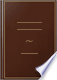Books Books 1 - 10 of 51 on If the same quantity, or equal quantities, be added to equal quantities, the sums....If the same quantity, or equal quantities, be added to equal quantities, the sums will be equal. 2. If the same quantity, or equal quantities, be subtracted from equal quantities, the remainders will be equal. 3. If equal quantities be multiplied by the...An Introduction to Algebra: With Notes and Observations: Designed for the ... - Page 3
by John Bonnycastle - 1782 - 201 pages## Cyclomathesis: Or, An Easy Introduction to the Several Branches of ..., Volume 4

William Emerson - Mathematics - 1764
..., ? , D , b, Si, í, X , &c. AXIOMS. 1. If equal quantities be added to equal quantities, the fums will be equal. 2. If equal quantities be taken from...quantities be multiplied by equal quantities, the produits will be equal. 4. If equal quantities be divided by equal quanties, the quotients will be...## An introduction to plain trigonometry, with its application to heights and ...

Richard Cockrel - 1792 - 99 pages
...'* ' SECTION 2. ..., A. XIOM S. " I. TF equal Quantities be added to equal J_ Quantities, their Sums will be equal. 2. If equal Quantities be taken from...equal Quantities, the Remainders will be equal. 3. If unequal Quantities be added to unequal Quantities, their Sums will be unequal. 4. If unequal Quantities...## The Elements of Geometry: In which the Principal Propositions of Euclid ...

William Emerson - Geometry - 1794 - 216 pages
...together. 2. If equal quantities be added to equal tjuank„. tities j the Tunis will be equal. i 3.* If equal quantities be taken from equal quantities ; the remainders will be equal. 4. If equal quantities be equally multiplied; the produfts will be equal. 5. If equal quantities be...## The Accomplished Tutor: Or, Complete System of Liberal Education ...

Thomas Hodson - Education - 1802
...Icndwri quantity. i. If equal quantities be added to eqiTdl quantities, the ftims will be equal. And if equal quantities be taken from equal quantities, the remainders will be equal. a. If equal quantities be multiplied by equal quantities, the produces will be equal. And if equal...## The Accomplished Tutor: Or, Complete System of Liberal Education ..., Volume 2

Thomas Hodson - Education - 1806
...quantity. Axioms. i. If equal quantities be added to equal quantities, the fums will be equal. And if equal quantities be taken from equal quantities, the remainders will be equal. 2. If equal quantities be multiplied by equal quantities, the products will be equal. And if equal...## The British Encyclopedia, Or Dictionary of Arts and Sciences ..., Volume 1

...deducted. AXIOMS. 1. If equal quantities be added to equal quantities, the sums will be equal. '.: If equal quantities be taken from equal quantities, the remainders will be equal. It is immaterial in what order the quantities are set down, if we take care to prefix to each its proper...## An Introduction to Algebra: Being the First Part of a Course of Mathematics ...

Jeremiah Day - Algebra - 1814 - 303 pages
...equal quantities, their sums will be equal. 2. If the same quantity or equal quantities be subtracted from equal quantities, the remainders will be equal. 3. If equal quantities be multiplied into the same, or equal quantities, tkeproduets will be equal. 4. If equal quantities be divided by...## American Edition of the British Encyclopedia: Or, Dictionary of ..., Volume 1

...the quantity 2 a A is deducted. AXIOMS. 1. If equal quantities be added to equal quantities, the sums will be equal. 2. If equal quantities be taken from...will be equal 3. If equal quantities be multiplied by the same, or equal quantities, the products will be equal. 4. If equal quantities be divided by the...## Geometrical Problems Deducible from the First Six Books of Euclid, Arranged ...

Miles Bland - Geometry - 1821 - 438 pages
...expressed. AXIOMS. (11.) If equal quantities be added to equal quantities, the sums will be equal. (12.) If equal quantities be taken from equal quantities, the remainders will be equal. (13.) If equal quantities be multiplied by the same or equal quantities,'the products will be equal....